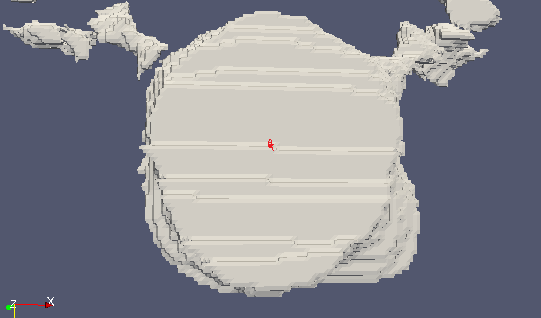# Calculate the centroid of a simplex surface?

I have simplex surface and I need to calculate centroid of different parts of the simplex? The edges are not similar in every side. Screenshots attached.For a closed volume part, one way to do it is to:

1. pick a random point as guessed center
2. calculate volume of each tetrahedron formed by the point and the triangles of the part
3. keep a sum of the multiples of small tetrahedons’ volume and center
4. divide the sum by the total volume

Here is an example code.

1 Like

This is simplex mesh. If I would say, may be all of the simplex cells will not have the same number of edges like 6 or 8. Still is it gonna work with your steps?

Calculating volume of a single cell formed by a single face and arbitrary center will be more complicated than a simple vector cross product, but it should still work.